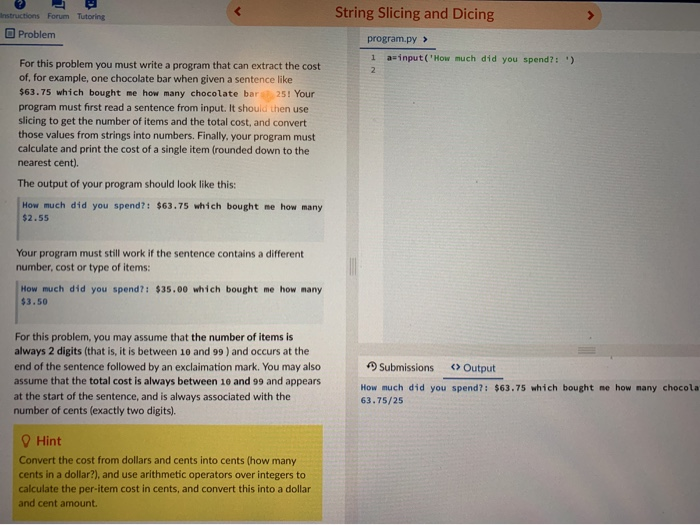### Create an Account

Home / Questions / a=input(How much did you spend?:) For this proble" aria-describedby="eor" style="

# a=input(How much did you spend?:) For this proble" aria-describedby="eor" style="a=input(How much did you spend?:) For this proble" aria-describedby="eor"> Instructions Forum Tutoring String Slicing and Dicing Problem program.py > a=input(&#39;How much did you spend?:) For this problem you must write a program that can extract the cost of, for example, one chocolate bar when given a sentence like \$63.75 which bought me how many chocolate bar 25! Your program must first read a sentence from input. It should then use slicing to get the number of items and the total cost, and convert those values from strings into numbers. Finally, your program must calculate and print the cost of a single item (rounded down to the nearest cent). The output of your program should look like this: How much did you spend?: \$63.75 which bought me how many \$2.55 Your program must still work if the sentence contains a different number, cost or type of items: How much did you spend?: \$35.00 which bought me how many \$3.50 For this problem, you may assume that the number of items is always 2 digits (that is, it is between 10 and 99 ) and occurs at the end of the sentence followed by an exclaimation mark. You may also assume that the total cost is always between 10 and 99 and appears at the start of the sentence, and is always associated with the number of cents (exactly two digits). Submissions <> Output How much did you spend?: \$63.75 which bought me how many chocola 63.75/25 Hint Convert the cost from dollars and cents into cents (how many cents in a dollar?), and use arithmetic operators over integers to calculate the per-item cost in cents, and convert this into a dollar and cent amount.

Apr 02 2020 View more View LessSubscribe To Get Solution